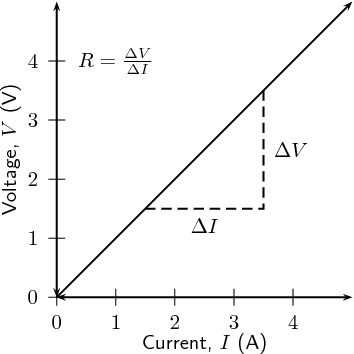Verification of Ohm's Law

Measurement of resistance.

## Objective :

To study the dependence of current (I) on the potential difference (V) across a resistor and determine its resistance. Also plot a graph between V and I.

## Material required :

An unknown resistance coil or a resistance wire, a voltmeter and a ammeter of appropriate( 1.5 V ) range, a battery, a rheostat, one way key , connecting wires and a piece of sand paper.

### Concept of Resistance:

Resistance is the property of a component which restricts the flow of electric current. Energy is used up as the voltage across the component drives the current through it and this energy appears as heat in the component.
Resistance is measured in ohms, the symbol for ohm is an omega(Ω).

Resistance can be both good and bad. If we are trying to transmit electricity from one place to another through a conductor, resistance is undesirable in the conductor. Resistance causes some of the electrical energy to turn into heat so some electrical energy is lost along the way. .

However, it is resistance that allows us to use electricity for heat and light. The heat that is generated from electric heaters or the light that we get from light bulbs is due to resistance. In a light bulb, the electricity flowing through the filament, or the tiny wires inside the bulb, cause them to glow white hot. If all the oxygen were not removed from inside the bulb, the wires would burn up.

### Resistors connected in Series :

When resistors are connected in series their combined resistance is equal to the individual resistances added together. For example if resistors R1 and R2 are connected in series their combined resistance, R, is given by:
Combined resistance in series:   R = R1 + R2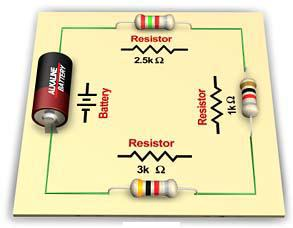### Resistors connected in parallel :

When resistors are connected in parallel their combined resistance is less than any of the individual resistances. There is a special equation for the combined resistance of two resistors R1 and R2: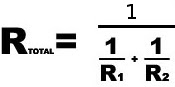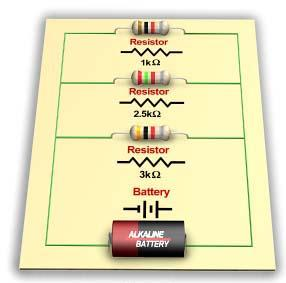How do we read resistor values :

The resistance of resistors is indicated using colour-coded bands on the body of the resistor. The first three colour bands indicate the value of the resistor in Ohms. The first band tells us the first digit, the second band tell us the second digit and the third band tell us the number of zeros.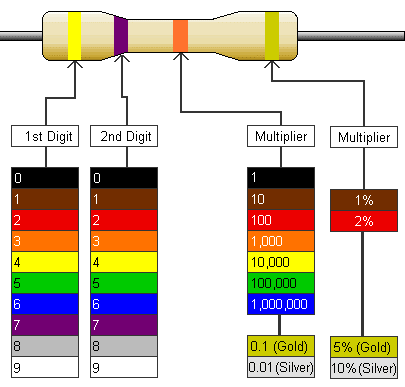### Example :

If the first band is green (5) the second digit is blue (6) and the third band is orange (3), the value of the resistor is 56000 ohm. Because 1000 Ohm = 1 KΩ, we have 56kΩ

## Ohm's Law :

If I be the current flowing through a conductor and V be the potential difference across its ends, then according to Ohm's Law,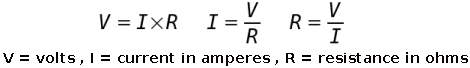### Circuit Diagram :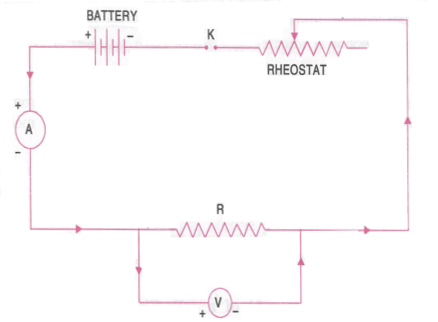### Graph :

If we plot voltage on the x-axis of a graph and current on the y-axis of the graph, we will get a straight-line. The gradient of the straight-line graph is related to the resistance of the conductor.